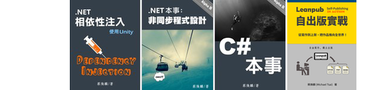從 C# 9 開始，需要自訂型別的時候，除了類別（class）、結構（struct），現在多了一個選擇：記錄（record）。其主要用途是封裝資料，特別是不可改變的資料（immutable data）。

```public record Student
{
public int Id { get; set; }
public string Name { get; set; }
}```

```var stu = new Student { Id=1, Name="Mike" };
stu.Id = 10;```

`public record Student (int Id, string Name);`

`Student stu1 = new (1, "Mike");`

```Student stu1 = new (1, "Mike") { Id=2 }; // OK!
Student stu2 = new ();              // 編譯失敗!
Student stu3 = new () { Id=3 };     // 編譯失敗! ```

```public record Student (int Id, string Name)
{
protected int Id { get; init; }
public string Name { get; } = Name;
public int Grade { get; init; }
}```

• 第 3 行改寫了參數列的 `Id` 屬性，而且把那個屬性的可見範圍限縮為 `protected`
• 第 4 行改寫了參數列的 `Name` 屬性，而且把它改成唯讀屬性。請注意這裡的初始值會由參數列（即建構式）的 `Name` 參數傳入。儘管同一個區塊中出現三個 `Name`，乍看之下可能產生誤解，但編譯器並不會搞錯。
• 第 5 行只是單純加入一個屬性。

```record ExStudent(int Id, string Name, int Grade)
: Student(Id, Name);```

`public record struct Student(...);`

### 編譯器產生的程式碼

`public record Student (int Id, string Name);`

### 複製物件

`<Clone>\$` 可以在 .NET 中介語言（IL）層次正常運作，但我們寫程式的時候是沒辦法呼叫它的（即便它是個公開方法）。那麼，編譯器什麼時候會替我們呼叫這個方法呢？

```var stu1 = new Student(1, "Mike");
var stu2 = stu1;             // 不會呼叫 <Clone>\$()
var stu3 = stu1 with { };      // 會呼叫 <Clone>\$()
var stu4 = stu1 with { Id=2 }; // 會呼叫 <Clone>\$()```

1. 首先，`<Clone>\$` 方法透過拷貝建構式來複製出一個新的副本。此複製過程是單純的一對一複製，不會去執行屬性的 `init` 存取子；亦即把來源記錄中的所有屬性和欄位（包括私有欄位）全部複製到新的記錄（副本）。
2. 接著會執行 `with` 關鍵字後面的成員初始設定式（member initializer），以便修改副本的屬性。

```var stu2 = new Student(stu1); // 使用拷貝建構式來完全複製
stu2.Id = 2;                  // 更新屬性值```

### `ToString` 方法

```public class MyClass
{
public int Id { get; set; }
public int Name { get; set; }
}

public record Student (int Id, string Name);```

```MyClass obj = new() { Id=1, Name="Mike" };
Console.WriteLine(obj.ToString());

Student stu = new (1, "Mike");
Console.WriteLine(stu.ToString());```

``````MyClass
Student { Id = 1, Name = Mike }
``````

### `Equals` 方法

```MyClass obj1 = new() { Id=1, Name="Mike" };
MyClass obj2 = new() { Id=1, Name="Mike" };
Console.WriteLine(\$"兩物件是否相等: {obj1.Equals(obj2)}");

Student stu1 = new (1, "Mike");
Student stu2 = new (1, "Mike");
Console.WriteLine(\$"兩物件是否相等: {stu1.Equals(stu2)}");```

``````兩物件是否相等: False

``````

```class Student : IEquatable<Student>
{
public override bool Equals(object? obj)
{  return Equals(obj as Student); }

// 實作 IEquatable<T> 介面的 Equals 方法
public virtual bool Equals(Student? other)
{ // 比對各屬性是否相等的程式碼（省略） }

protected virtual Type EqualityContract
{ get => typeof(Student); }
}```

1. 宣告型別的地方（第 1 行），編譯器把 `record` 變成了 `class`，而且實作了 `IEquatable<T>` 介面，以便比對兩個物件是否相等。
2. 第 3 行是改寫 `Object` 的 `Equals` 方法，其內部只是去呼叫 `IEquatable<T>` 介面的 `Equals` 方法（第 7 行）。

1. 兩物件的參考是否相等。若相等則傳回 True。
2. 兩物件的 `EqualityContract` 是否相等。從剛才程式碼列表的倒數第 3 行可得知，這裡比較的是兩物件是否為同一個型別。若型別相等，才繼續往下比較。
3. 逐一比較兩物件的所有屬性和欄位（包括私有欄位）。若全部相等便傳回 True，否則傳回 False。

```// 兩個物件的內容完全相同，但型別不同。
MyClass obj = new() { Id=1, Name="Mike" };
Student stu = new (1, "Mike");
Console.WriteLine(stu.Equals(obj)); // False!```

### `Deconstruct` 方法

```class Student : IEquatable<Student>
{
public void Deconstruct(out int Id, out string Name)
{
Id = this.Id;
Name = this.Name;
}
// 其餘省略
}```

```Student stu = new (1, "Mike");

var (id, name) = stu;
Console.WriteLine(\$"id={id}, name={name}");```

``````id=1, name=Mike
``````

### 結語

• 可用簡短的語法寫出內含多項常用功能的物件，包括內建的複製操作和 `ToString` 方法、比較兩個物件的內容是否相同、分解式等等。
• 容易設計出唯讀物件，故特別適合用來封裝不可變的（immutable）資料物件。